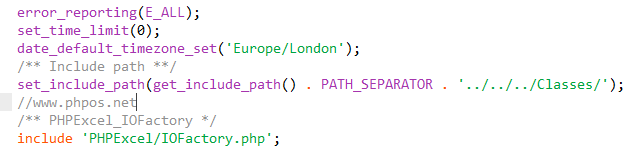# DEDE批量导入excel数据到后台文章系统的开发教程

1）开发前的准备：2) 开发插入数据代码：

 1 2 3 4 5 6 7 8 9 10 11 12 13 14 15 16 17 18 19 20 21 22 23 24 25 26 27 28 29 30 31 32 33 34 35 36 37 38 39 40 41 42 43 44 45 46 47 48 49 50 51 52 53 54 55 56 57 58 59 60 61 62 63 64 65 66 67 68 69 70 71 72 73 74 75 76 77 78 79 80 81 82 83 84 85 86 87 88 89 90 91 92 93 94 95 96 97 98 99 100 101 102 103 104 105 ``` ``` ``` header( "Content-type:text/html;charset=utf8" ); require_once ( '/../dedecms/include/common.inc.php' ); error_reporting (E_ALL); set_time_limit(0); date_default_timezone_set( 'Europe/London' ); /** Include path **/ set_include_path(get_include_path().PATH_SEPARATOR . '../../../Classes/' ); /** PHPExcel_IOFactory */ include 'PHPExcel/IOFactory.php' ; if (! empty ( \$_GET )){ \$typeid = \$_GET [ 'typeid' ]; \$dopost = \$_GET [ 'do' ]; if ( \$dopost == "exdata" ){ if (! empty ( \$_GET [ 'n' ])){ \$inputFileName = './' . \$_GET [ 'n' ]. '.xlsx' ; \$objPHPExcel = PHPExcel_IOFactory::load( \$inputFileName ); \$sheetData = \$objPHPExcel ->getActiveSheet()->toArray(null,true,true,true); \$rowarr = array (); \$dsql ->Execute( 'all' , "select bio2,bio1 from `dede_addonshop`" ); while ( \$rowall = \$dsql ->GetArray( 'all' )) { \$rowarr []= \$rowall ; } foreach ( \$sheetData as \$v ) { foreach ( \$rowarr as \$vt ) { if (in_array( \$v [ 'B' ], \$vt )&& \$v [ 'A' ]== \$vt [ 'bio1' ]){ echo "货号为：" . \$v [ 'B' ]. "厂商为：" . \$v [ 'A' ]. "的数据已在表中,请在excel文件里面删除这一条后再添加！" ; exit ; } } } //附加表插入数据前处理 \$row = \$dsql ->GetOne( "select aid,bio2 from `dede_addonshop` order by aid desc" ); if (! empty ( \$row )){ \$aid = \$row [ 'aid' ]; \$bio2 = \$row [ 'bio2' ]; } else { \$aid = 0; \$bio2 = '' ; } //处理重复问题 if ( \$bio2 == \$sheetData [ count ( \$sheetData )][ 'B' ]){ ShowMsg( "不能重复添加内容" , 'javascript:;' ); exit ; } //主表插入数据前处理 \$arcrow = \$dsql ->GetOne( "select id from `dede_archives` order by id desc" ); if (! empty ( \$arcrow )){ \$arcid = \$arcrow [ 'id' ]; } else { \$arcid = 0; } //微表插入数据前处理 \$tinyrow = \$dsql ->GetOne( "select id from `dede_arctiny` order by id desc" ); if (! empty ( \$tinyrow )){ \$tinyid = \$tinyrow [ 'id' ]; } else { \$tinyid = 0; } //找出最大的id \$id = max( \$aid , \$arcid , \$tinyid ); \$alphalpha = array ( 'A' , 'B' , 'C' , 'D' , 'E' , 'F' , 'G' , 'H' , 'I' , 'J' , 'K' , 'L' , 'M' , 'N' , 'O' , 'P' , 'Q' , 'R' , 'S' , 'T' , 'U' , 'V' , 'W' , 'X' , 'Y' , 'Z' , 'AA' , 'AB' , 'AC' , 'AD' , 'AE' , 'AF' , 'AG' , 'AH' , 'AI' , 'AJ' , 'AK' , 'AL' , 'AM' , 'AN' , 'AO' , 'AP' , 'AQ' , 'AR' , 'AS' , 'AT' , 'AU' , 'AV' , 'AW' , 'AX' , 'AY' , 'AZ' ); //字段数量 \$fieldnum = count ( \$sheetData ); \$fields = \$fieldvalue = '' ; for ( \$i =0; \$i < \$fieldnum ; \$i ++) { \$fields .= \$sheetData [ \$alphalpha [ \$i ]]. ',' ; } \$fields = substr ( \$fields ,0,-1); //遍历数组 foreach ( \$sheetData as \$value ) { \$pubdate = GetMkTime(GetDateTimeMk(time())); \$click = mt_rand(50,200); if ( \$value [ 'A' ]== 'bio1' | \$value [ 'A' ]== '厂商' ){ continue ; } \$id = \$id +1; //获取字段值\$value['A']; for ( \$i =0; \$i < \$fieldnum ; \$i ++) { \$fieldvalue .= " ,'" . \$value [ \$alphalpha [ \$i ]]. "' " ; } //标题 \$C = trim( \$value [ 'C' ]); //保存到主表 \$senddate = time(); \$arcquery = "INSERT INTO `dede_archives`(id,typeid,title,mid,channel,pubdate,senddate,click,ismake)VALUES ('\$id','\$typeid','\$C','1','6','\$pubdate','\$senddate','\$click','-1');" ; \$dsql ->ExecuteNoneQuery( \$arcquery ); //保存到附近加表 \$query = "INSERT INTO `dede_addonshop`(aid,typeid, \$fields ) VALUES ( '\$id' , '\$typeid' { \$fieldvalue });"; \$dsql ->ExecuteNoneQuery( \$query ); \$fieldvalue = '' ; //保存到微表 \$tinyquery = "INSERT INTO `dede_arctiny`(id,typeid,channel,mid,senddate)VALUES ('\$id','\$typeid','6','1','\$senddate');" ; \$dsql ->ExecuteNoneQuery( \$tinyquery ); } \$num = count ( \$sheetData )-2; ShowMsg( "恭喜,成功插入   " . \$num . "   条数据！" , 'javascript:;' ); } } } else { echo "密码或文件名错误！您无权做任何操作！" ; } ?> ``` ` `

1）第一步先判断问号后面是不是为空,即dopost=exdata&typeid=6&n=test1字符串是否为空,如果这个字符串都是空的话,其它,就不用操作了,直接通过出程序,显示：echo "密码或文件名错误！您无权做任何操作！";

 1 2 `\$typeid` `=` `\$_GET` `[` `'typeid'` `];` `\$dopost` `=` `\$_GET` `[` `'do'` `];`

3）插入数据库表时最大数据id的判断

3）遍历数组\$sheetData插入数据

# 浏览历史

18luck手机客户端下载